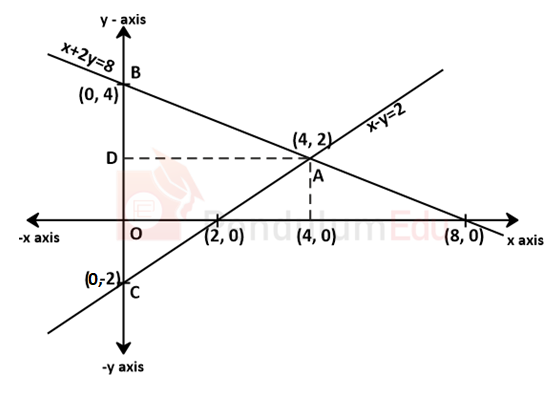Question of The Day20-12-2021

Find the area of the triangle formed by the straight line x – y = 2 , x + 2y = 8 and y- axis.

Correct Answer : a ) 12 sq. unit

Explanation :

According to the question

Straight lines are:

x – y = 2……..(1)

x + 2y = 8…….(2)

x = 0……….(3)

Now to find the area of the triangle, we need to find the coordinates of the vertices of the triangle.

Point at which line 1 and 2 intersect:

Subtracting eq(1) form eq (2)

⇒ 3y = 6

⇒ y = 2

⇒ x – y = 2

⇒ x = 2 + 2

⇒ x = 4

⇒ Both the lines intersect at (4, 2)

Point at which line 1 and 3 intersect:

x = 0 and y = -2

Point at which line 2 and 3 intersect:

x = 0 and y = 4As shown in figure

Area of △ABC

Base (BC) = 4 + 2

⇒ 6 units

Area of triangle=$${1 \over 2}$$*base*height

$${1 \over 2}*6*4$$

⇒ 12 sq. units

Hence, (a) is the correct answer.0